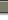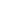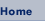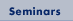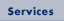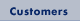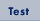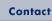Joglekar Associates Inc.
2820 Fountain Lane North
Minneapolis, MN 55447
Ph: 763-476-1449
Fx: 763-475-3823Test

Just for fun, here are some multiple-choice test questions. Green belts, black belts and technical people using statistical methods should be able to answer such questions. For each question there is only one correct answer. Good luck!

1. A doctor prescribed two tablets per day for a patient. The drug content of each tablet has a normal distribution with mean = 50 mg and standard deviation = 4 mg. What is the standard deviation of drug taken by the patient per day?

1. 4
2. 2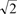3. 44. 8
5. 82. To compare two treatments in terms of their mean, what is the approximate sample size per treatment if the difference in mean to be detected is equal to the standard deviation, the alpha-risk is 5% and the beta-risk is 10%?

1. 10
2. 20
3. 30
4. 35
5. 40

3. Regression analysis was used to obtain the following equation relating response Y to input factors A and B.

Y = 100 + 3A – 0.5B + error

The % confidence associated with both coefficients in the equation exceeded 95% and the R-square value turned out to be 20%. Which of the following statements is not true?

1. B has a small but statistically significant effect on Y
2. Measurement error must be small to find this small effect of B
3. A has a statistically significant effect on Y
4. Some other factor is influencing Y
5. Measurement error may be large

4. Why do variable charts occur in pairs (e.g. X-bar and R) and attribute charts occur singly (e.g. p)?

1. Variable data are more precise
2. Both mean and variance can be computed for variable data but not for attribute data
3. Variance is a deterministic function of mean for the attribute data
4. Attribute data have only two categories, knowing one you know the other
5. None of the above

5. Given the same data, five students computed the values of Cp, Cpk, Pp and Ppk indices. Which computations are likely to be correct?

 Cp Cpk Pp Ppk a 2 1.5 1.33 1.0 b 1.5 1.7 1.42 1.0 c 2.0 1.0 0.62 0.5 d 1.82 1.0 1.47 1.2 e Both a and c above

6. From an X-bar chart, variance within subgroup was found to be 2 and variance between subgroups was found to be 0.1. What action should be taken to significantly improve the process?

1. Eliminate time trend
2. Eliminate shift-to-shift differences
3. Supplier needs to improve batch-to-batch variability
4. Measurement system variability should be evaluated
5. Remove cycling along the time axis

7. A and B are fixed factors in a two-level factorial experiment, the two levels of each factor being –1 and +1. Analysis of the factorial experiment resulted in the following equation

Y = 10 + 4A + 3B + error

What is the variance component due to factor A?

1. 40
2. 83. 12
4. 4
5. 16

8. A hamburger chain had five fast food restaurants. A different group of ten randomly selected people was sent to each restaurant (a total of 50 people) and each person evaluated the quality of two hamburgers. What is the structure for this data collection scheme?

1. Restaurants and people are crossed and hamburgers are nested
2. All three variables are crossed
3. People and hamburgers are crossed but are nested inside restaurants
4. Hamburgers are nested inside people who are nested inside restaurants
5. None of the above

9. If W represents half the width of a two-sided specification, to achieve six sigma quality, the standard deviation of measurement should be less than which of the following?

1. W
2. W/2
3. W/4
4. W/8
5. W/12